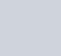Which among the following is the strongest acid?
Question:

Which among the following is the strongest acid?

1. $\mathrm{CH}_{3} \mathrm{CH}_{2} \mathrm{CH}_{2} \mathrm{CH} 3$

2.3.4.Correct Option: , 4

Solution: